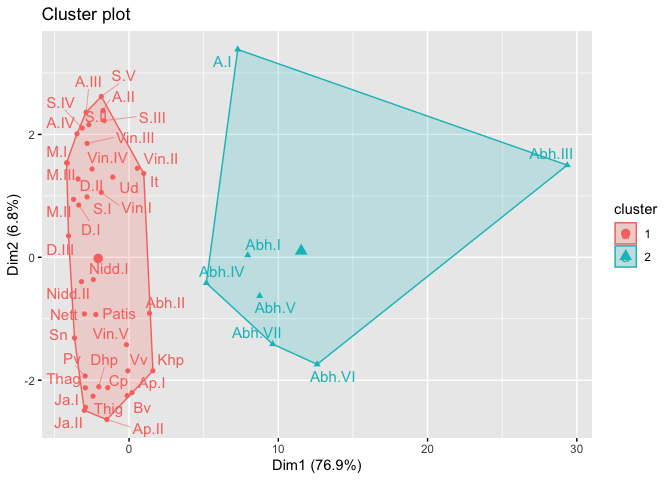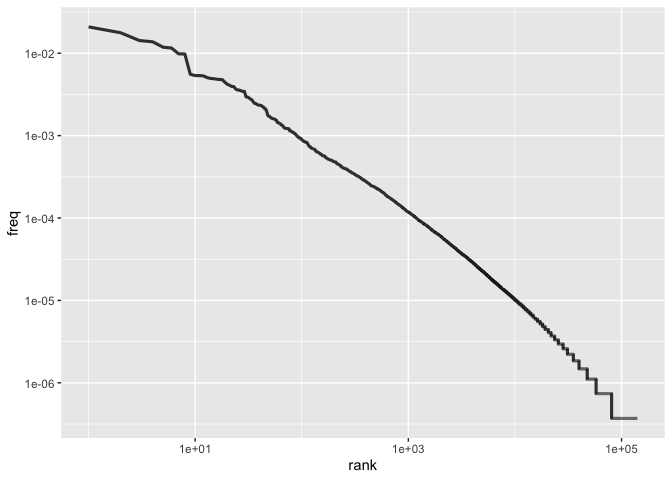# tipitaka

The goal of tipitaka is to allow students and researchers to apply the tools of computational linguistics to the ancient Buddhist texts known as the Tipitaka or Pali Canon.

The Tipitaka is the canonical scripture of Theravadin Buddhists worldwide. It purports to record the direct teachings of the historical Buddha. It was first recorded in written form in what is now Sri Lanka, likely around 100 BCE.

The tipitaka package primarily consists of the texts of the Tipitaka in various electronic forms, plus a few simple functions and data structures for working with the Pali language.

The version of the Tipitaka included here is based on what’s known as the Chattha Sangāyana Tipiṭaka version 4.0 (aka, CST4) published by the Vipassana Research Institute and received from them in April 2020. I have made a few edits to the CST4 files in creating this package:

• Where volumes were split across multiple files, they are here are combined as a single volume

• Where volume numbering was inconsistent with the widely-used Pali Text Society (PTS) scheme, I have tried to conform with PTS.

• A very few typos that were found while processing have been corrected.

There is no universal script for Pali; traditionally each Buddhist country ususes its own script to write Pali phonetically. This package uses the Roman script and the diacritical system developed by the PTS. However, note that the Pali alphabet does NOT follow the alphabetical ordering of English or other Roman-script languages. For this reason, tipitaka includes `pali_alphabet` giving the full Pali alphabet in order, and the functions, `pali_lt`, `pali_gt`, `pali_eq`, and `pali_sort` for comparing and sorting Pali strings.

## Installation

You can install the released version of tipitaka from CRAN with:

``install.packages("tipitaka")``

And the development version from GitHub with:

``````# install.packages("devtools")
devtools::install_github("dangerzig/tipitaka")``````

## Example

You can use tipitaka to do clustering analysis of the various books of the Pali Canon. For example:

``````library(tipitaka)
dist_m <- dist(tipitaka_wide)
cluster <- hclust(dist_m)
plot(cluster)``````You can also create traditional k-means clusters and visualize these using packages like `factoextra`:

``````library(factoextra) # great visualizer for clusters
km <- kmeans(dist_m, 2, nstart = 25, algorithm = "Lloyd")
fviz_cluster(km, dist_m, labelsize = 12, repel = TRUE)``````You can also explore the topics of various parts of the Tipitaka using packges like `wordcloud`:

``````library(wordcloud)
library(dplyr)
sati_sutta_long %>%
anti_join(pali_stop_words, by = "word") %>%
with(wordcloud(word, n, max.words = 40)) ``````Finally, we can look at word frequency by rank:

``````library(dplyr, quietly = TRUE)
freq_by_rank <- tipitaka_long %>%
group_by(word) %>%
add_count(wt = n, name = "word_total") %>%
ungroup() %>%
distinct(word, .keep_all = TRUE) %>%
mutate(tipitaka_total =
sum(distinct(tipitaka_long, book,
.keep_all = TRUE)\$total)) %>%
transform(freq = word_total/tipitaka_total) %>%
arrange(desc(freq)) %>%
mutate(rank = row_number()) %>%
select(-n, -total, -book)

freq_by_rank %>%
ggplot(aes(rank, freq)) +
geom_line(size = 1.1, alpha = 0.8, show.legend = FALSE) +
scale_x_log10() +
scale_y_log10()``````